upm  1.7.1 Sensor/Actuator repository for libmraa (v2.0.0)
LM35 Class Reference

API for the DFRobot LM35 Linear Temperature Sensor. More...

## Detailed Description

This sensor returns an analog voltage proportional to the temperature of the ambient environment.

It has a range of 2C to 150C.

This driver was developed using the DFRobot LM35 Linear Temperature Sensor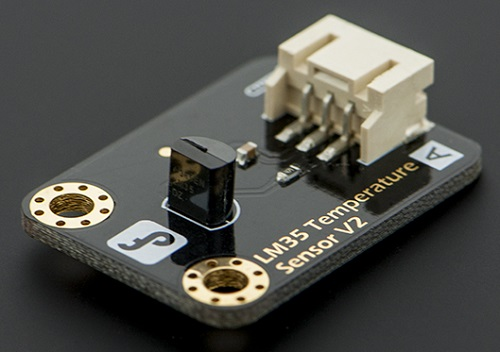// Instantiate a LM35 on analog pin A0, with a default analog
// reference voltage of 5.0
upm::LM35 sensor(0);
// Every half second, sample the sensor and output the temperature
while (shouldRun) {
cout << "Temperature: " << sensor.getTemperature() << " C" << endl;
upm_delay_us(500000);
}

## Public Member Functions

LM35 (int pin, float aref=5.0)

~LM35 ()

float getTemperature ()

void setScale (float scale)

void setOffset (float offset)

## Protected Attributes

lm35_context m_lm35

## Constructor & Destructor Documentation

 LM35 ( int pin, float aref = `5.0` )

LM35 constructor

Parameters
 pin Analog pin to use aref Analog reference voltage; default is 5.0 V
 ~LM35 ( )

LM35 destructor

Here is the call graph for this function: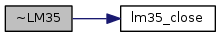## Member Function Documentation

 float getTemperature ( void )

Returns the temperature in degrees Celsius

Returns
The Temperature in degrees Celsius

Here is the call graph for this function: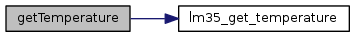void setScale ( float scale )

Set sensor scale. This scale is applied to the return values before the offset is applied. Default is 1.0.

Parameters
 scale Scale to apply.

Here is the call graph for this function: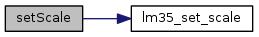void setOffset ( float offset )

Set sensor offset. This offset is applied to the return values before scaling. Default is 0.0.

Parameters
 offset Offset to apply.

Here is the call graph for this function: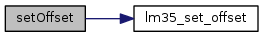Collaboration diagram for LM35: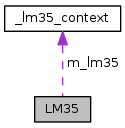[legend]

The documentation for this class was generated from the following files: